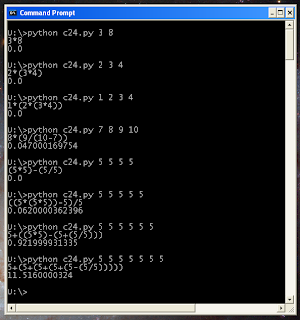## 6/29/06

### "calculate 24" python implementation

“Calculate 24″ is one of my childhood game. It was played like this: 2 kids pull 4 cards from a stack of playing cards and try to get the number 24 out of them，he who calculate correctly first wins.

The rules are:
1, Each card must be used once and only once.
2, One can only use addition, subtraction, multiplication, and division.
3, the intermediate result must be integer.

For example: 1 3 3 10 -> (10+1-3)*3=24

The 3rd rule are often violated by almost all other implementations out there on the web, which give solution like (5-1/5)*5=24 or 6/(1-3/4)=24. It's good for generate brain teasers, but it's not valid. And these algorithms (often use floating point calculation) that ignoring 3rd rule are actually much easier to program.

Here is the program.Some note:
Function bags() gets the bags of a list, in each bag, the list is divided into 2 parts. for example: bags([1,2,3,4] will give us:

[,[2,3,4]], [[1,2],[3,4]], [[1,2,3],]

calrec() is the recursive engine that do the calculation on a list. From each bags of a list, it perform + - * / operation on the 2 parts. Each part will also call calrec() on itself. In the end, we get all combinations of calculations on a list.

calrec() only calculate on an ordered list. We need to do this on all permutation of the list. The function permu() gets the permutations of a list without redundant items, see the previous post.

We call permu() in calc(), where we call calrec() on each permutation. Whenever we see result 24, we return.

The program can not only be used on 4 numbers, it can take any numbers (>1). For example:
# python c24.py 3 8
3*8
0.000813961029053
# python c24.py 5 5 5 5 5
((5*(5*5))-5)/5
1.08426403999

Of course, with growing numbers, the execution time grow exponentially.

The program can also be easily called on any expected number, not just 24.

(This blog is moved here from my old blog)